The K5 Learning Blog urges parents to be pro-active in helping their children reach their full academic potential.

K5 Learning
provides free worksheets, workbooks and an online reading and math program for kindergarten to grade 5 students.# How to subtract fractions with like denominators

## What are fractions?

A fraction is a part of a whole.  Every fraction has a denominator and a numerator.

## Denominator

The bottom number of the fraction is called the denominator. It shows how many equal parts the item is divided into.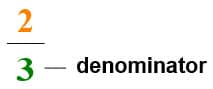## Numerator

The number above the line shows how many parts we have.

##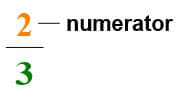How to subtract fractions with like denominators

There are three steps to subtract fractions with like denominators.

1.    Make sure the denominators are the same. Let’s show you in an example: ¾ - ¼.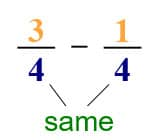2.    Subtract the numerators.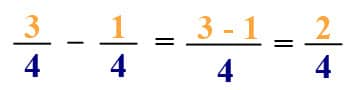3.    Simplify the fraction (if needed).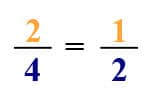In an earlier blog about adding fractions, we described how to simplify fractions. If you need to review how to simplify fractions, you can read about that here.

## Practice worksheets

if you want to practice subtracting fractions, try these grade 5 grade worksheets.